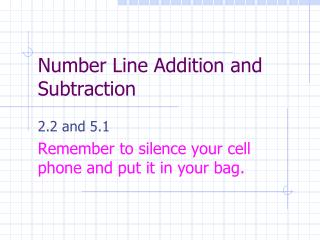DownloadDownload PresentationTélécharger la présentation- - - - - - - - - - - - - - - - - - - - - - - - - - - E N D - - - - - - - - - - - - - - - - - - - - - - - - - - -
Presentation Transcript

1. Number Line Addition and Subtraction 2.2 and 5.1 Remember to silence your cell phone and put it in your bag.

2. Whole Number Addition a + b = ? • Start at 0, facing the positive direction. • Move forward a. • Move forward b.

3. Whole Number Subtraction a – b = ? • Start at 0, facing the positive direction. • Move forward a. • Turn around. • Move forward b.

4. Integer Addition a + b = ? • Start at 0, facing the positive direction. • If a>0, move forward a. If a<0, move backward |a|. • If b>0, move forward b. If b<0, move backward |b|.

5. Integer Subtraction a – b = ? • Start at 0, facing the positive direction. • If a>0, move forward a. If a<0, move backward |a|. • Turn around. • If b>0, move forward b. If b<0, move backward |b|.

6. Modeling Integer Operations I do not have to model integer operations the same way Dr. Simons does, BUT I must get my model approved prior to Test 1.